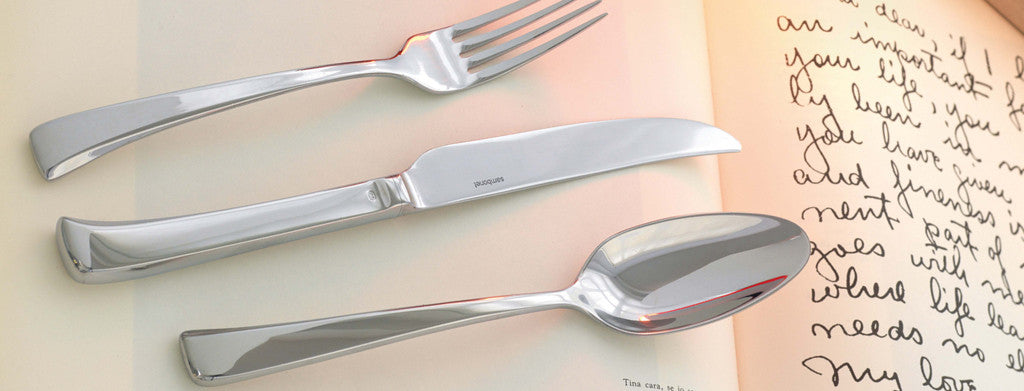Shopping Cart

### Refine

View all

#### Price# Cutlery£11.77£0.29 each (ex VAT)£0.50 each (ex VAT)£7.36 each (ex VAT)£7.36 each (ex VAT)£10.51 each (ex VAT)£10.51 each (ex VAT)£13.38£13.38£0.32 each (ex VAT)£0.29 each (ex VAT)£0.95 each (ex VAT)£0.50 each (ex VAT)£0.48 each (ex VAT)£0.48 each (ex VAT)£0.89 each (ex VAT)£0.48 each (ex VAT)£0.53 each (ex VAT)£1.18 each (ex VAT)£7.36 each (ex VAT)£10.51 each (ex VAT)£13.52£13.52£13.38 each (ex VAT)£14.76 each (ex VAT)£10.75 each (ex VAT)£12.01 each (ex VAT)£12.88£12.88£12.47 each (ex VAT)£0.50 each (ex VAT)£0.95 each (ex VAT)£0.50 each (ex VAT)£0.95 each (ex VAT)£0.48 each (ex VAT)£0.48 each (ex VAT)£0.89 each (ex VAT)£0.48 each (ex VAT)£0.32 each (ex VAT)£0.32 each (ex VAT)£0.50 each (ex VAT)£0.95 each (ex VAT)£0.42 each (ex VAT)£1.10 each (ex VAT)£1.10 each (ex VAT)£1.10 each (ex VAT)£0.94 each (ex VAT)£1.19 each (ex VAT)